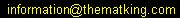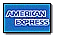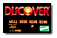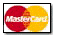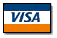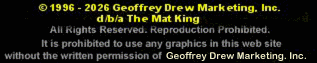DRIVE TENSION CALCULATIONS FOR STRAIGHT RUNNING BELTS

### (Consult factory for TURN BELTS)

#### Important

• Drive tension is used to determine the maximum load a belt can handle without premature fatigue and failure.
• Consult Keystone for application assistance when approaching the maximum tension or for complex systems, as well as for the maximum number of sprockets that can be used for a given belt width.
• The figures for the maximum allowable tension are given for drum-driven applications. In order for the belt to withstand these tensions with a sprocket-driven system, it is necessary to place a sprocket in every drive opening.

Use the following equation for rough calculations. This calculation CANNOT be used for Key-Turn belts.

1. Determine the drive tension (Td) as shown below:
Td = (F x B x L) (2WB + WL)
Where:
 Td = Drive Tension (lbs.) WB = Weight of Belt (lbs/ft2) WL = Weight of Load on Belt (lbs/ft2) F = Friction Factor (see Table, below) B = Belt Width (ft.) L = Conveyor Length (ft.) (1/2 the belt length)
1. Calculate the drive tension per foot of belt width by dividing Td by the belt width (B).
2. If using the belt at an elevated temperature, multiply the maximum allowable tension per foot of width (given in the conveyor specifications tables, pages 2-6), by a factor from the table below to get the working tension at an elevated temperature.
3. Compare the calculated value from step 2 with the maximum allowable tension found in step 3. The calculated value cannot exceed the maximum allowable tension.

Back to Convayor Belting Index

Back to Index

THE MAT KING
740 West End Avenue; Suite 1
New York, NY 10025 USA

Phone: (646)998-4208
Fax: (646)998-4073
Phone: 800-442-6544
Fax: 800-Mats-Fax (628-7329)

Phone: 800-442-6544
Fax: 800-Mats-Fax (628-7329)